# Logarithm

Computing logarithms is the inverse of exponentiation, as subtraction is the inverse of addition and division is the inverse of multiplication. For example, since 343 = 73, the base-7 logarithm of 343 is 3. In general, if a = bc then logb(a) = c, where b is called the base and c is the logarithm. In other words, the logarithm c is the exponential power to which the base must be raised to produce the number a.

Logarithms show up in a variety of scientific formulas: for example, the number of bits required to represent the address of a particular location in computer memory is equal to the base-2 logarithm (rounded upwards) of the number of possible locations. Logarithms are also useful for representing large numbers in a more manageable form.

##  Logarithms of intermediate and fractional values

Because we use a base-10 number system, it is often convenient to use 10 as the base of the logarithm. It's easy to find the base-10 logarithm of a number such as 1000, because 1000 = 103, therefore log10(1000) = 3. (The value of the logarithmic function is the number that appeared as an exponent in the other equation.) But what is the logarithm of a number such as 43, or 0.001?

We know from the study of exponents that, for example,$10^\frac{1}{2}$ is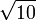$\sqrt{10}$ and this then supplies a value for$\log_{10}(\sqrt{10}) = 0.5$. Values for many other numbers can be worked out similarly using cube roots and so on, and values for all real numbers can then be defined using limits. For example, log10(43) is approximately equal to 1.63347. Multiplying a number by 10 appends a zero to the numeral (if it is an integer) and adds 1 to the base-10 logarithm, therefore log10(430) is approximately 2.63347.$10^{-3} = \frac{10}{10^4} = 0.001$

and it then follows that

log10(0.001) = − 3,

or in general,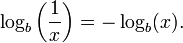$\log_b\left(\frac{1}{x}\right) = - \log_b(x).$

By a similar argument it can be established that b0 = 1 for any base b > 1 and therefore that logb(1) = 0.

Thus, for any base b > 1 logarithms of numbers between 0 and 1 are negative numbers, the logarithm of 1 is 0, and logarithms of numbers greater than 1 are positive real numbers as seen in the image below.

##  Shape of the logarithm function

Consider the function f(x) = logb(x) where b is a real-number base greater than 1 of the logarithm. Since any positive real number raised to the exponent zero is 1, the logarithm of 1 is zero. To the right of 1 on the x-axis, the function f continually increases, but increases more and more slowly as x heads towards infinity. Between 1 and 0, the logarithmic function has negative values, and asymptotically approaches minus infinity as x approaches zero. For negative values of x, there is no defined value of f(x) within the real numbers — but using complex numbers a value can be found, as will be discussed further below.

##  Manipulating logarithms

Suppose we know the logarithm of a number using one base b, and we want to find the logarithm using a different base c :

logb(x) = y
logc(x) = ?

Suppose we know y, b and c and we want to find logc(x). We need to look up the value of logc(b), and then by multiplying we can find the desired quantity:

logc(x) = logc(b)logb(x)

This formula can be established using the definition of logarithms and the rule for multiplying exponents: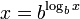$x = b^{\log_bx}$$b = c^{\log_cb}$

Substituting for b,$x = (c^{\log_cb})^{\log_bx}$$x = c^{\log_c(b) \log_b(x)}$

Therefore logc(x) = logc(b)logb(x), the formula we wished to prove.

A useful formula for log(xy) can be derived using the rule for adding exponents: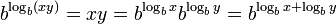$b^{\log_b(xy)} = xy = b^{\log_bx}b^{\log_by} = b^{\log_bx + \log_by}$

Therefore :

logb(xy) = logb(x) + logb(y)

Other useful formulas include these identities:

logb(x / y) = logb(x) − logb(y)
logb(xy) = ylogb(x)$\log_b (\sqrt[y]{x}) = \frac{\log_b (x)}{y}$

## Bases and notational variants

The base must not be 0 nor 1. The most widely used bases for logarithms are 10, 2 and the transcendental number e (also known as Euler's number) which is approximately 2.7182818284.

When logarithms are used to measure physical values (e.g., when noise is measured in decibels) it is common to use 10 as a base, and in these contexts, the notation$\log \, x$ means$\log_{10} \,x$.

Mathematicians and physicists often find, however, that e is more convenient as a base for a logarithmic function. The logarithmic function with e as a base is called the natural logarithm; it has the special property that its derivative is 1 / x. The notation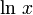$\ln \, x$ is often used for natural logarithms, but in mathematics it is just as common to use the notation$\log\, x$ and write logarithms to base 10 using the full notation$\log_{10}\, x$.

Computer science adds a whole new wrinkle because, in some cases, it is most useful to consider logarithms to base 2, and this has led to a new notation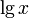$\mathrm{lg}\,x$ which become common for logarithms to a base of 2. Finally, the notation$\log\, x$ is sometimes used for logarithms to base 2, but this is usage is less common.

In German and Russian literature, lg is used to mean the common logarithm log10(x). The advice of the United States Department of Commerce's National Institute of Standards and Technology is to follow the ISO standard Mathematical signs and symbols for use in physical sciences and technology, ISO 31-11:1992, which suggests these notations:

• The notation ln(x) means loge(x)
• The notation lg(x) means log10(x)
• The notation lb(x) means log2(x)

It is obvious that, until a standard such as the ISO standard is universally accepted, perhaps the surest way to avoid confusion is to always use the notation of loge(x), log10(x), or log2(x), whichever is applicable.

## Complex numbers and logarithms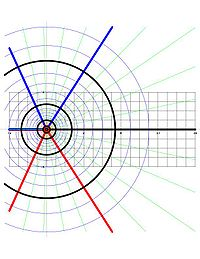$~f=\ln(z)~$ with lines$~\Re(f)=\rm const~$ and lines$~\Im(f)=\rm const~$ in the complex$~z~$-plane.

The exponential function of an imaginary number is given as$e^{ix} = \cos(x) + i\sin(x).\,$

Consequently if u and v are real numbers then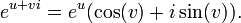$e^{u + vi} = e^u(\cos(v) + i\sin(v)).\,$

The sine and cosine functions are not one-to-one and hence neither is this complex exponential function. Therefore it has no inverse function, as the concept of "function" is generally defined in the present day. However, one may speak of the logarithm as being a multiple-valued function (strictly speaking, an oxymoronic term by current conventions).

To find the logarithm of a complex number a + bi, it is convenient to express the number in polar coordinates (r,θ) where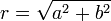$r = \sqrt{a^2 + b^2}$ and θ = arctan(b / a). (Intuitively, r is the length of the line segment joining the number to the origin in the complex plane, and θ is the angle that line segment makes with the x-axis). The equivalence of the two notations is given by$a + bi = r(\cos(\theta) + i \sin(\theta)).\,$

Let us define c + di so that ln(a + bi) = c + di. Using the formula for the exponential function above,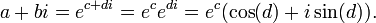$a + bi = e^{c + di} = e^ce^{di} = e^c(\cos(d) + i \sin(d)).\,$

It can be seen from similarity with the above formula for polar coordinates that r = ec and d = θ. Therefore,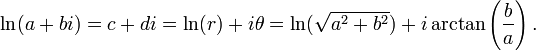$\ln(a + bi) = c + di = \ln( r ) + i \theta = \ln(\sqrt{a^2 + b^2}) + i \arctan\left(\frac{b}{a}\right).\,$

This is "multiple-valued" because the arctangent function is "multiple-valued". If w is a value of ln(a + bi), then w + 2nπi could be also considered a value of the "function" for any integer n. However, in numerical calculus, it is preferable to work with a function that returns number rather than a set of possible values. In the case of multivalued functions, the result of evaluation of a function cannot be used for evaluation of another function; in addition, in the case of logarithm, the set of values is infinite. Intents to work with multivalued functions as if they would be "conventional" functions leads to confusions. Therefore, usually one works with single-valued functions.

A single-valued logarithmic function is defined by, restricting the values of the arctan function to$-\pi < \theta \le \pi$. In this way, the log function is interpreted in the most of programming languages - Mathematica, Maple(software), Matlab, C++; evaluation of the function log returns value f with$-\pi < \Im(f)\le \pi$.

In this way, the domain of the logarithmic function can be extended to cover the entire complex plane except for the number zero, which has an undefined value — a singularity with the real part plunging towards minus infinity and the imaginary part spinning wildly like a compass at the North Pole. The function is discontinuous along the negative x-axis (see figure); this line of discontinuity is called the cut of the logarithm. The logarithm is a holomorphic function on the complex plane except for the origin and the cut.

## History

The method of logarithms was first publicly propounded in 1614, in a book entitled Mirifici Logarithmorum Canonis Descriptio, by John Napier, Baron of Merchiston, in Scotland.

Jost Bürgi, a Swiss mathematician, independently discovered logarithms but did not publish his discovery until four years after Napier.

In 1617, the English mathematician, Henry Briggs, after collaborative discussions with Napier, published his first tables of logarithms using a base of 10 rather than Napier's original base of 1/e. In 1624, Briggs published his Arithmetica Logarithmica containing the logarithms of thirty thousand numbers to fourteen decimal places (1 to 20,000 and 90,000 to 100,000). To this day, logarithms using the base ten are often referred to as "Briggsian" logarithms.

Early resistance to the use of logarithms was muted by the support of Johannes Kepler, the German mathematician and astronomer, who published a clear and impeccable explanation in 1624 of how logarithms worked.

The use of logarithms contributed to the advance of science, and especially of astronomy, by making some difficult calculations possible. Prior to the advent of electronic calculators and computers, logarithms were used extensively in surveying, navigation, engineering, chemistry and many other disciplines.

##  The importance of logarithms

The development of electronic calculators and computers in the mid-1900's reduced the importance of logarithms for computations but not the importance of logarithmic functions. Thus, we should discuss the importance of logarithms before and after the advent of electronic calculators and computers.

###  Before the advent of calculators and computers

The operations of addition and subtraction are much easier to perform than are the operations of multiplication and division. Logarithms were characterized by Pierre-Simon Laplace, the French mathematician and astronomer, as "doubling the life of an astronomer". The German mathematician, Karl Friedrich Gauss, who also did work in physics and astronomy, is said to have memorized a table of logarithms to save the time required to look up a logarithm each time he needed one.

The use of logarithms was widespread because of their relative simplicity compared to multiplication, division, or raising numbers to an exponential power. A few numerical examples, using base-10 logarithms (to eight decimal places) will illustrate that simplicity.

Example 1: Calculate 112.76 × 3,085.31 by using logb(xy) = logb(x) + logb(y):

log10(112.76) + log10(3085.31) = 2.05215507 + 3.48929881 = 5.54145387
antilog10(5.54145387) = 347,899.55

The answer would be 347,899.56 by using an electronic calculator.

Example 2: Calculate 47.53 ÷ 860.22 by using logb(x / y) = logb(x) − logb(y):

log10(47.53) − log10(860.22) = 1.67696781 − 2.93460954 = −1.25764172
antilog10(−1.25764172) = 0.05525233

The answer would be 0.05525233 by using an electronic calculator.

Example 3: Calculate 963.641/3 using logb(xy) = ylogb(x):

(1/3) × log10(963.64) = (1/3) × 2.98391482 = 0.99463827
antilog10 (0.99463827) = 9.87730064

The answer would be 9.87730064 by using an electronic calculator.

Note: The antilog of x is simply the logarithm base raised to the power of x which, in the above examples, is 10x.

###  After the advent of calculators and computers

Although calculators and computers have essentially replaced the use of logarithms for arithmetic computations (multiplication, division, finding roots, exponentiation, etc.), they are still used for various purposes in many fields. For example:

• In chemistry, the acidity or alkalinity of a solution is expressed in terms of the pH scale and pH is defined as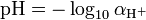$\mbox{pH} = -\log_{10} \alpha_{\mathrm{H}^+}$  where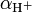$\alpha_{\mathrm{H}^+}$ is the activity of dissolved hydrogen ions in the solution.
• In the field of seismology, the magnitude of seismic events such as earthquakes are measured on a logarithmic scale. Each whole number increase in magnitude represents a tenfold increase in the amplitude of the earthquake. In terms of energy, each whole number increase corresponds to an increase of about 31.6 times the energy released. Each two number increase corresponds to about 1000 times the energy released.
• A great many sets of scientific data are correlated by plotting the data in graphs that use one or two axes that are logarithmic (referred to as semi-log or log-log graphs).